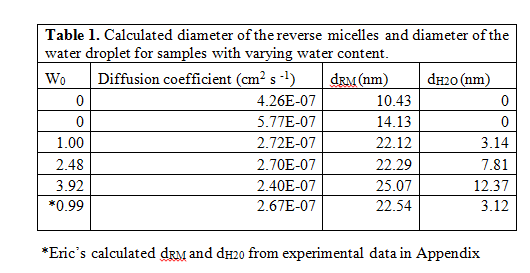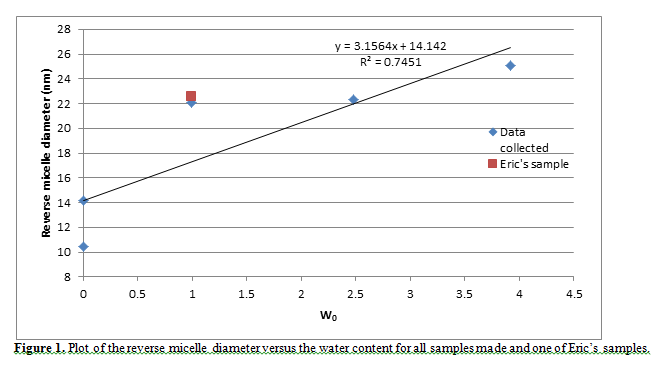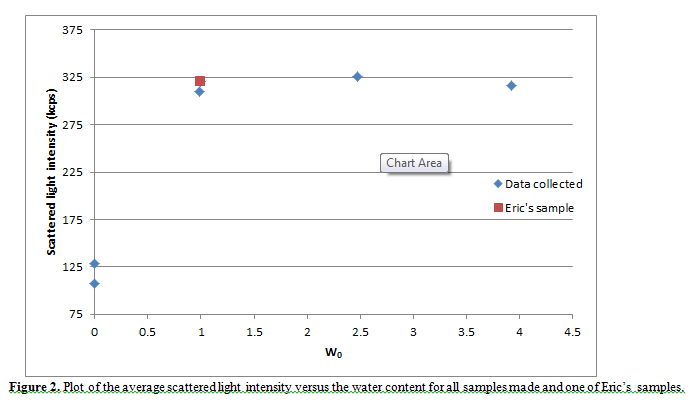# Dynamic Light Scattering: Reverse Micelle Size Dependence on Water Content

By: Scott Holdren

Abstract

Dynamic light scattering is used to measure the size of a reverse micelle by determining the diffusion coefficient and average intensity of scattered light of various solutions. These solutions are made using the surfactant Triton X-100 in cyclohexane and varying amounts of water. As more water is added, the size of the reverse micelle increases which increases the scattering of the light from the laser. For the various solutions made, the diameter of the micelle ranges from 10-25 nm and the diameter of the water dropletranges from 3-12 nm.

1. Introduction

The purpose of the experiment is to use dynamic light scattering as a technique to find the size of Triton X-100 reverse micelles which vary based on the amount of water in each micelle. The size of these molecular aggregates increases as more water is added to the surfactant. The diameter (dRM) is calculated by determining the diffusion coefficient of each micelle using the DLS instrument. In addition, the DLS instrument measures the average intensity of scattered light for each sample which increases with the amount of water content (W0).

2. Experimental

The experimental steps were followed as described on the handout. The experimental data was combined with my lab partner and a total of five samples at 35 °C were made and analyzed using dynamic light scattering. The exact W0 values, volume of water added, diffusion coefficient, and average intensity of scattered light can be found in the Appendix attached to the report (Table 2). In addition to the data listed above, there were some additional experimental parameters recorded. The wavelength and angle of the laser were set at 632.8 nm and 90°, respectively. Also, the first time delay was recorded as 1.0 μs and the last delay as 10.0 ms.

3.  Results and discussion

To calculate the reverse micelle diameter, the diffusion coefficient is used along with the viscosity coefficient of cyclohexane at 35 °C (η = 0.75 mPa*s) found in Lange et al.The reverse micelle diameter is found by calculating the hydrodynamic radius of the micelle using the Stokes-Einstein equation and multiplying this value by two. The reverse micelle diameter (dRM) with the corresponding diffusion coefficient obtained from each sample can be found in Table 1.Next, the diameter of the reverse micelle versus the water content was plotted and is shown in Figure 1. The relationship between the size and water content of the micelle was expected to be linear. However, Figure 1 shows that there is uncertainty in the linear fit that is applied to the data set. Notice that data was also taken from Eric’s sample (W0 = 0.99) to verify if the W0 =1.00 sample we made was an outlier and could be ignored. However, since the two W0 samples appeared to calculate approximately the same dRM, our sample could not be ignored. The slope of the best fit line (m) is 3.156 and from the equation in the handout, the slope can be used to find the diameter of the water droplet (dH2O) in each sample by dH2O = m (W0).  Table 1 on the previous page lists the calculated diameter of the water droplets for each of the five samples.One reason why the relationship between the water content and the size of the reverse micelle is not linear could be that the equation dRM = dH20 + 2 lc is not an accurate, mathematical description for the reverse micelles analyzed. For example, the length of the hydrophobic chain of Triton X-100 (lc) in the equation is assuming that for each reverse micelle, the length of the hydrophobic chains will always be equal to lc. However, the hydrophobic chains could form coils around one another and could be shorter or longer in any given reverse micelle. This would cause fluctuations in the light scattered and would affect the calculated dRM.

Next, the scattered light intensity versus water content was plotted in Figure 2. The trend observed in this plot appears to be logarithmic rather than the predicted linear relationship. One could expect the plot to be linear because the size of the reverse micelle is directly related to the amount of light scattered. As the micelle becomes larger, more of the light that passes through the sample will be redirected from these larger aggregates.

However, Figure 2 shows that as the water content in the micelle increases, the scattered light will increase in a logarithmic manner. This could possibly be explained from the variable size of the micelles. At a certain point, although the size of the water droplet increases, it is possible that the scattered light may only increase very slightly. To test this, one would need to use the DLS instrument to measure more samples of W0 > 4 and see if the scattered light continues to increase in a logarithmic fashion or increases dramatically.1. Summary

The experiment showed that dynamic light scattering is a useful method to determine the size of a reverse micelle using Triton X-100 as the surfactant. Although there is uncertainty in the data obtained and calculated, one can observe that as the amount of water added increases, the size of the micelle (diameter of the micelle) also increases. In addition, since the plot shown in Figure 1 is not linear, the equation dRM = dH20 + 2 lc is not an accurate representation of the diameter of the micelle or the water droplet. This is because the length of the hydrophobic tails of the surfactant may vary in length for an individual micelle; therefore, it would affect the calculated diameter of the reverse micelle.

1. References
1. Lange, N. A.; Dean, J.A. Lange’s Handbook of Chemistry 1967, 10th ed. pp 1669-1674
1. Appendix
• Share